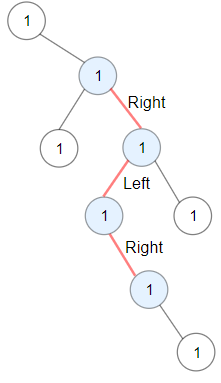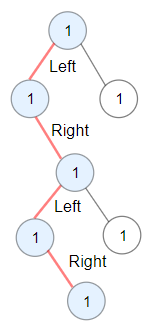# 1372. Longest ZigZag Path in a Binary Tree ⭐

Medium
You are given the `root` of a binary tree.
A ZigZag path for a binary tree is defined as follow:
• Choose any node in the binary tree and a direction (right or left).
• If the current direction is right, move to the right child of the current node; otherwise, move to the left child.
• Change the direction from right to left or from left to right.
• Repeat the second and third steps until you can't move in the tree.
Zigzag length is defined as the number of nodes visited - 1. (A single node has a length of 0).
Return the longest ZigZag path contained in that tree.
Example 1:Input: root = [1,null,1,1,1,null,null,1,1,null,1,null,null,null,1,null,1]
Output: 3
Explanation: Longest ZigZag path in blue nodes (right -> left -> right).
Example 2:Input: root = [1,1,1,null,1,null,null,1,1,null,1]
Output: 4
Explanation: Longest ZigZag path in blue nodes (left -> right -> left -> right).
Example 3:
Input: root = 
Output: 0
Constraints:
• The number of nodes in the tree is in the range `[1, 5 * 10^4]`.
• `1 <= Node.val <= 100`

### 解題

/**
* Definition for a binary tree node.
* type TreeNode struct {
* Val int
* Left *TreeNode
* Right *TreeNode
* }
*/
func longestZigZag(root *TreeNode) int {
ans := 0
var helper func(*TreeNode, int, bool)
helper = func(root *TreeNode, length int, goLeft bool) {
if root == nil { return }
ans = max(length, ans)
if goLeft {
helper(root.Left, length + 1, false) // 前面的長度加上這一條
helper(root.Right, 1, true) // 應該要往左走卻往右走，步數歸零在+1
} else {
helper(root.Right, length + 1, true)
helper(root.Left, 1, false)
}
}
helper(root, 0, true)
helper(root, 0, false)
return ans
}
func max(a, b int) int {
if a > b { return a }
return b
}